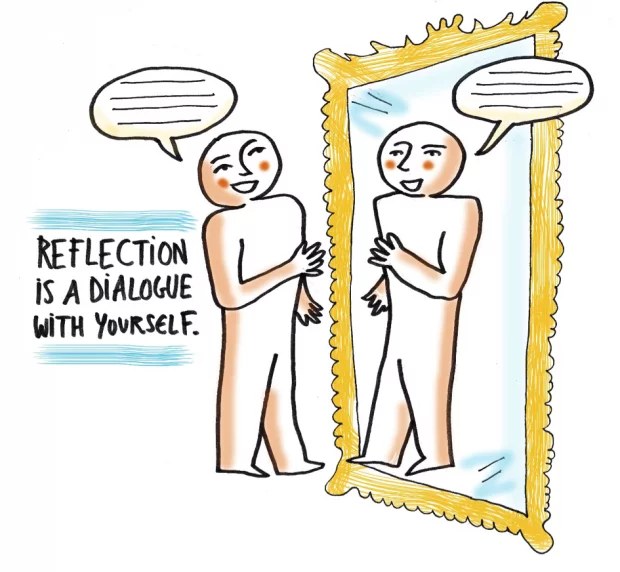Select Page

# Overflow & Rounding

based on resources from code.org

## Food for thought

### Objective:

This unit introduces students to the practical aspects of using a binary system to represent numbers in a computing device. Students discover the limitations of creating numbers that are “too big” or “too small” to count. They learn that, while a number system is infinite, the physical representation of numbers requires place values — which are finite, and limit the ability to represent numbers.

Food for thought

Imagine you going to the supermarket, you have four Rs.100 notes, nine Rs.10 notes and one Rs.5 coin.

1. Whats the most expensive item you can purchase?
2. Whats the least expensive item you can purchase without getting any change back?
3. What would you do if your friend needs Rs. 7 ?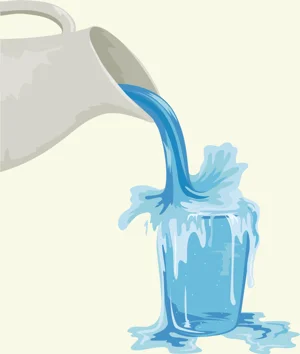## Overflow & rounding

We will start exploring large place values to see what happens when a big number gets too big. Go to the Binary Odometer Widget.

Explore the odometer to understand how it works.

Set the odometer to the highest number possible. Then let it run!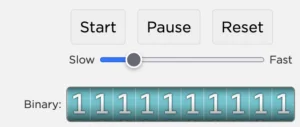What happened to the odometer reading? Is the reading of odometer still valid?

## Flippydo pro

Now that you have started thinking about place value and overflow, we are going to work on a different problem. What happens when there aren’t enough place values to represent a number? You will explore this with a new version of the Flippy Do, the Flippy Do Pro!

Make the smallest nonzero number possible, in binary, with your Flipply Do Pro.

What is the decimal (Base 10) equivalent?

Increase the number made in Task 1 to the next value with your Flipply Do Pro.

What is the decimal equivalent? (What comes after 000000.01  ? )

Make the binary equivalent of 0.75

Can you make the binary number for 0.39 ?

What is the largest number (in decimal) you can make with the Flippy Do Pro?

# PIE CHALLENGE

Use a Flippy Do Pro to determine how much pie is left at the end of dessert. For each pie, make a decision how you want to round the number to fit on the Flippy Do Pro.

### Pie #1 – Pumpkin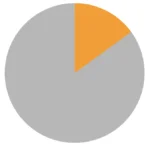Do this:

1. Estimate how big the orange slice is.
2. Use your Flippy Do Pro to represent how big the orange slice is.  You may need to round up or down.
3. Convert your binary number to a decimal number.
4. Write down the number in your journal

### Pie #2 – Cherry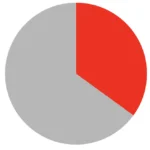Do this:

1. Estimate how big the red slice is.
2. Use your Flippy Do Pro to represent how big the red slice is.  You may need to round up or down.
3. Convert your binary number to a decimal number.
4. Write down the number in your journal.

### Pie #3 – Lemon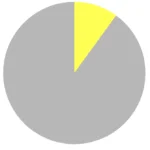Do this:

1. Estimate how big the yellow slice is.
2. Use your Flippy Do Pro to represent how big the yellow slice is.  You may need to round up or down.
3. Convert your binary number to a decimal number.
4. Write down the number your journal.

### Pie #4 – Chocolate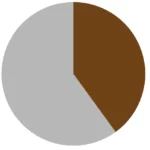Do this:

1. Estimate how big the brown slice is.
2. Use your Flippy Do Pro to represent how big the Brown slice is.  You may need to round up or down.
3. Convert your binary number to a decimal number.
4. Write down the number your journal.

How much pie is left?
Add all the decimal numbers up together.

Check with others in Google Classroom and discuss.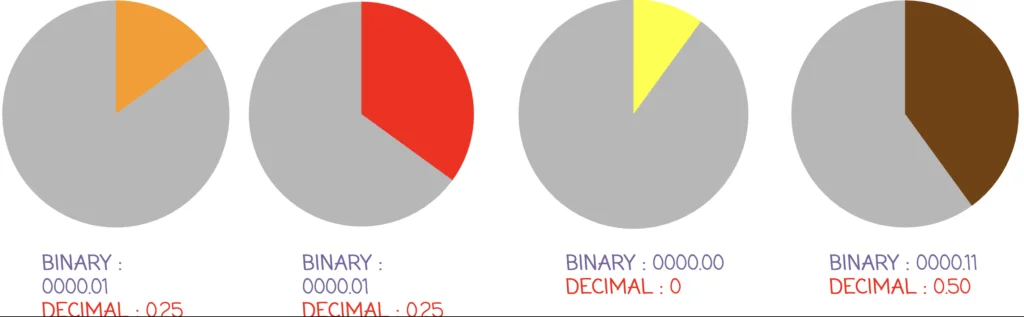or like this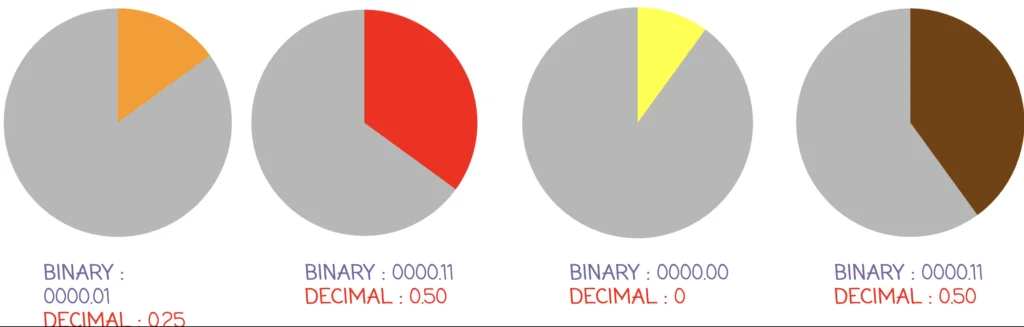Your answer may be a little different depending on how you rounded – up or down. If you did not have to round at all, you would see that exactly one whole pie is left over!

Why is it a problem for a computer if your answers are different than others?

## Reflection

What does the binary odometer show about representing large numbers?

What does the Flippy Do Pro show about representing very small numbers?

If we had a big enough odometer or Flippy Do Pro, could we represent every possible number?

Modern car odometers record up to a million miles driven. What happens to the odometer reading when a car drives beyond its maximum reading?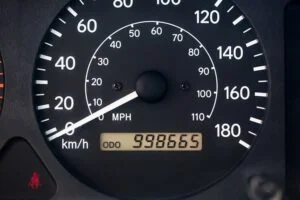When using bits to represent fractions of a number, can you create all possible fractions? Why or why not?